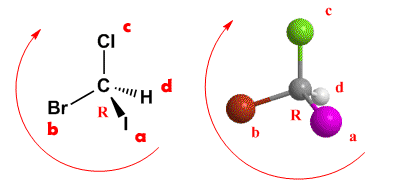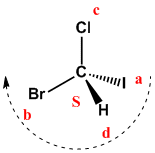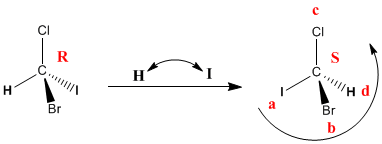A nomenclature is necessary that distinguishes the stereoisomers of a molecule. Thus, in the case of 2-Chlorobutane the notation must distinguish one enantiomer from the other. Cahn, Ingold and Prelog developed some rules that allow us to distinguish some stereoisomers from others, which I describe below.

Rule 1.- Give priorities to each of the four atoms or groups of atoms directly linked to the asymmetric carbon. This priority is given by atomic number. In the Bromochloroiodomethane molecule, iodine is the group with the highest priority, which we represent by “a” and hydrogen is the group with the lowest priority, which we represent by “d”.Rule 2.- Orient the molecule with the lowest priority group towards the bottom of the paper. In the previous example, the group with the lowest priority is hydrogen (group d) and it is as can be seen in the figure at the bottom of the paper (dashed line). Now we draw an arrow that goes through the groups in order a → b → c. If the travel is clockwise, the asymmetrical carbon has the notation R. If the travel is counterclockwise, the carbon configuration is S.R/S notation of molecule and image
Note that if an enantiomer has the notation R, its mirror image will be S.R/S notation with wedge "d" group
In the above examples the d group always lies behind the plane formed by the three highest priority substituents. If the d group is towards us (wedge) the configuration of the carbon is contrary to the spin. Let's see an example:In this case the lowest priority group is oriented towards us. Priorities are given, but the chiral center notation is anti-spin. So, we rotate clockwise, but the notation is S.

R/S notation with group of in the plane
How do we give the absolute configuration of a chiral center that has the lowest priority group in the plane? One solution is to rotate the molecule to leave "d" at the bottom. But we can also use a "trick" that allows us to know in a simple way the absolute configuration of a chiral center. This idea is based on changing the group d by the group that goes to the bottom. A change of two groups involves changing the notation of the chiral center.Changing hydrogen to iodine changes the notation of the molecule. After making the change, the chiral center has the notation S. Therefore, the starting molecule will be R.